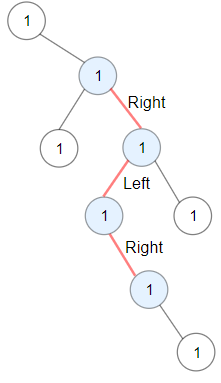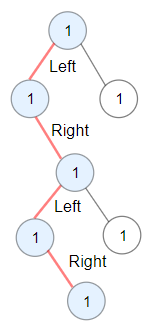# GeetCode Hub

You are given the `root` of a binary tree.

A ZigZag path for a binary tree is defined as follow:

• Choose any node in the binary tree and a direction (right or left).
• If the current direction is right, move to the right child of the current node; otherwise, move to the left child.
• Change the direction from right to left or from left to right.
• Repeat the second and third steps until you can't move in the tree.

Zigzag length is defined as the number of nodes visited - 1. (A single node has a length of 0).

Return the longest ZigZag path contained in that tree.

Example 1:```Input: root = [1,null,1,1,1,null,null,1,1,null,1,null,null,null,1,null,1]
Output: 3
Explanation: Longest ZigZag path in blue nodes (right -> left -> right).
```

Example 2:```Input: root = [1,1,1,null,1,null,null,1,1,null,1]
Output: 4
Explanation: Longest ZigZag path in blue nodes (left -> right -> left -> right).
```

Example 3:

```Input: root = 
Output: 0
```

Constraints:

• The number of nodes in the tree is in the range `[1, 5 * 104]`.
• `1 <= Node.val <= 100`

/** * Definition for a binary tree node. * public class TreeNode { * int val; * TreeNode left; * TreeNode right; * TreeNode() {} * TreeNode(int val) { this.val = val; } * TreeNode(int val, TreeNode left, TreeNode right) { * this.val = val; * this.left = left; * this.right = right; * } * } */ class Solution { public int longestZigZag(TreeNode root) { } }